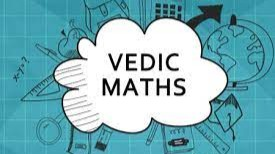# Vedic Maths - Fast Addition of multiple small numbers.

By ac05 | Vedic Maths | 2 May 2021

Ten Point Circle :-

There are only 10 digits that make up all infinite numbers. These are 0,1,2,3,4,5,6,7,8,9. You can make infinite possible numbers from them.. There is 5 numbers pair for digits to make 10. Lets visualize it.

1+9 =10              2+8 =10             3+7 =10          4+6 =10       5+5 =10

Use these 5 pairs to make group of "10" while adding numbers.

Lets solve a problem using this. Add 24+23+36+27.

Now looking at this problem you can be able to picture what groups you should make. As we knw adding group of 10's is easy. So, 24 will pair up with 36 and 23 with 27 i.e.

24+36    +    23+27

60       +      50

110

Pretty simple.

Lets do some problems on your own.

• 24+68+32+66
• 45+76+68+55+22+36

Numbers close to 10's :- Here I am talking about 7,8,9 ending terms.

These numbers are close to 10 i.e adding small number we could make them 10 i.e.

9+1 =10     8+2 =10    7+3 =10

While adding some number to these numbers we could use this trick to make our calculation easy. So, lets add 49 +6.

So to become 50, 49 need 1. And that 1 will be provided by 6.

49 + 6

49+ 1 -1 +6

50 + 6 -1

50 + 5

55

Lets do some problem on your own.

• 78+4
• 49+9
• 77+8

Sum of 10 :-

There are eight unique groups of three numbers that add up to make 10. For example, 1+2+7 =10, 2+2+6=10, 2+3+5=10 and so on.

this comes in handy when you have list of numbers but you cant apply above two techniques to add numbers. Lets understand about it more with example  7+1+6+2+4

Now looking at those number you could easily make 1-pair satisfying the first trick. But for rest number this trick would come handy. As we knw 1+2=3 and3 and 7 make pair to sum up to 10. So,

(6+4) + (1+2+7)

10   +    10

20

Lets solve some on your own

• 18+3+2+17
• 7+8+9+2+3+5+3+1+2+3+7+9
• 18 + 13 + 14 + 23

The motive behind this Post is to make readers do calculations in their mind rather than using calculators. Not only it increases your mental power but also makes them independent of calculators for basic calculations.

Resourcesac05

A competitive Programmer exploring different domainsVedic Maths

Vedic mathematics consists of various techniques that makes calculations easy and fast. Well i will be posting some of the vedic maths tricks, made by ancient Indian mathematics. If you grasp those tricks you could easily do calculation in your mind. Now you won't need calculator every time to do addition or multiplication or squaring or even square rooting a number. At the end of each post I will be adding few questions that you must do with those tricks without using calculator. Have a nice day!Send a \$0.01 microtip in crypto to the author, and earn yourself as you read!

20% to author / 80% to me.
We pay the tips from our rewards pool.